# GPU Survival Toolkit for the AI age: The bare minimum every developer must know

### Why CPU Knowledge Is No Longer Enough

In today's AI age, the majority of developers train in the CPU way. This knowledge has been part of our academics as well, so it's obvious to think and problem-solve in a CPU-oriented way.

However, the problem with CPUs is that they rely on a sequential architecture. In today's world, where we are dependent on numerous parallel tasks, CPUs are unable to work well in these scenarios.

Some problems faced by developers include:

CPUs traditionally operate linearly, executing one instruction at a time. This limitation stems from the fact that CPUs typically feature a few powerful cores optimized for single-threaded performance.

When faced with multiple tasks, a CPU allocates its resources to address each task one after the other, leading to a sequential execution of instructions. This approach becomes inefficient in scenarios where numerous tasks need simultaneous attention.

While we make efforts to enhance CPU performance through techniques like multi-threading, the fundamental design philosophy of CPUs prioritizes sequential execution.

#### Running AI Models Efficiently

AI models, employing advanced architectures like transformers, leverage parallel processing to enhance performance. Unlike older recurrent neural networks (RNNs) that operate sequentially, modern transformers such as GPT can concurrently process multiple words, increasing efficiency and capability in training. Because when we train in parallel, it will result in bigger models, and bigger models will yield better outputs.

The concept of parallelism extends beyond natural language processing to other domains like image recognition. For instance, AlexNet, an architecture in image recognition, demonstrates the power of parallel processing by processing different parts of an image simultaneously, allowing for accurate pattern identification.

However, CPUs, designed with a focus on single-threaded performance, struggle to fully exploit parallel processing potential. They face difficulties efficiently distributing and executing the numerous parallel computations required for intricate AI models.

As a result, the development of GPUs has become prevalent to address the specific needs of parallel processing in AI applications, unlocking higher efficiency and faster computation.

#### How GPU Driven Development Solves These Issues

Massive Parallelism With GPU Cores

Engineers design GPUs with smaller, highly specialized cores compared to the larger, more powerful cores found in CPUs. This architecture allows GPUs to execute a multitude of parallel tasks simultaneously.

The high number of cores in a GPU are well-suited for workloads depending on parallelism, such as graphics rendering and complex mathematical computations.

We will soon demonstrate how using GPU parallelism can reduce the time taken for complex tasks.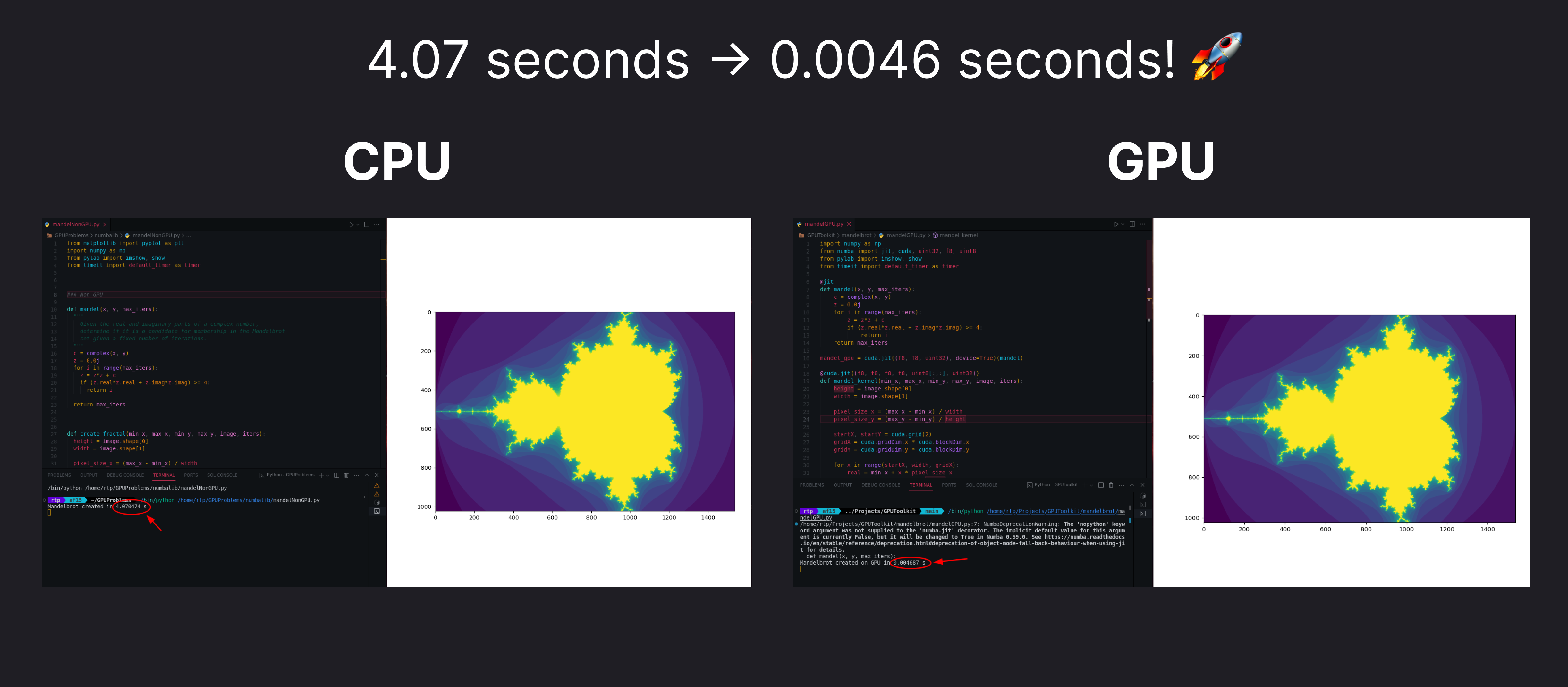Parallelism Used In AI Models

AI models, particularly those built on deep learning frameworks like TensorFlow, exhibit a high degree of parallelism. Neural network training involves numerous matrix operations, and GPUs, with their expansive core count, excel in parallelizing these operations. TensorFlow, along with other popular deep learning frameworks, optimizes to leverage GPU power for accelerating model training and inference.

We will show a demo soon how to train a neural network using the power of the GPU.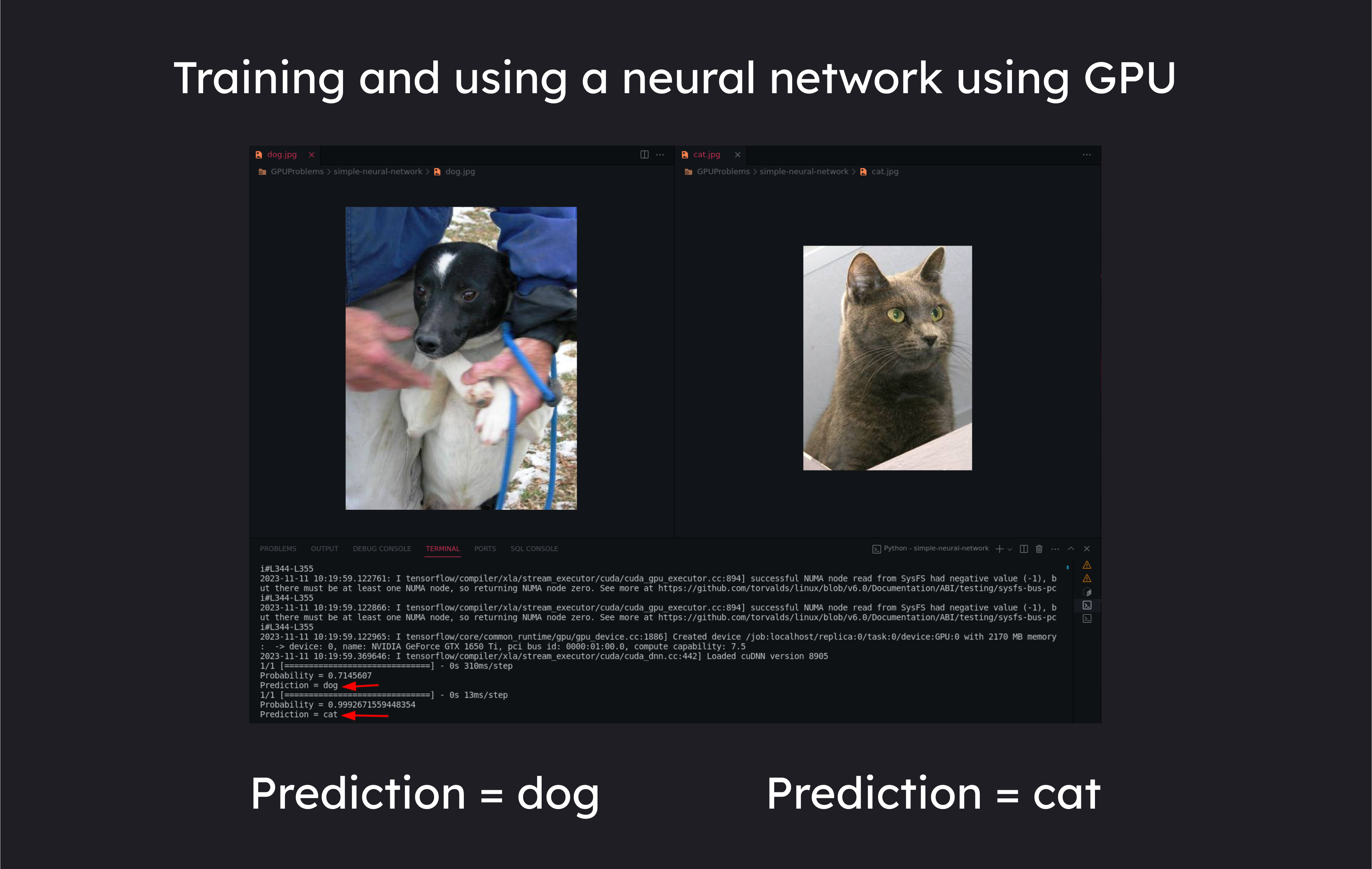### CPUs Vs GPUs: What’s the Difference?

#### CPU

Sequential Architecture

Central Processing Units (CPUs) are designed with a focus on sequential processing. They excel at executing a single set of instructions linearly.

CPUs are optimized for tasks that require high single-threaded performance, such as

• General-purpose computing
• System operations
• Handling complex algorithms that involve conditional branching

CPUs feature a smaller number of cores, often in the range of 2-16 cores in consumer-grade processors. Each core is capable of handling its own set of instructions independently.

#### GPU

Parallelized Architecture

Graphics Processing Units (GPUs) are designed with a parallel architecture, making them highly efficient for parallel processing tasks.

This is beneficial for

• Rendering graphics
• Performing complex mathematical calculations
• Running parallelizable algorithms

GPUs handle multiple tasks simultaneously by breaking them into smaller, parallel sub-tasks.

Thousands Of Cores For Parallel Tasks

Unlike CPUs, GPUs boast a significantly larger number of cores, often numbering in the thousands. These cores are organized into streaming multiprocessors (SMs) or similar structures.

The abundance of cores allows GPUs to process a massive amount of data concurrently, making them well-suited for parallelisable tasks, such as image and video processing, deep learning, and scientific simulations.

### AWS GPU Instances: A Beginner's Guide

Amazon Web Services (AWS) offers a variety of GPU instances used for things like machine learning.

Here are the different types of AWS GPU instances and their use cases:

General-Purpose Gpu Instances

• P3 and P4 instances serve as versatile general-purpose GPU instances, well-suited for a broad spectrum of workloads.

• These include machine learning training and inference, image processing, and video encoding. Their balanced capabilities make them a solid choice for diverse computational tasks.

• Pricing: The p3.2xlarge instance costs \$3.06 per hour.

• This provides 1 NVIDIA Tesla V100 GPU of 16 GB GPU memory

Inference-optimized GPU instances

• Inference is the process of running live data through a trained AI model to make a prediction or solve a task.

• P5 and Inf1 instances specifically cater to machine learning inference, excelling in scenarios where low latency and cost efficiency are essential.

• Pricing: the p5.48xlarge instance costs \$98.32 per hour.

• This provides 8 NVIDIA H100 GPUs of 80 GB memory each, totalling upto 640 GB Video Memory.

Graphics-optimized GPU instances

• G4 instances instances are engineered to handle graphics-intensive tasks.

• A video game developer might use a G4 instance to render 3D graphics for a video game.

• Pricing: g4dn.xlarge costs \$0.526 to run per hour.
• Uses 1 NVIDIA T4 GPU of 16 GB Memory.

Managed GPU Instances

• Amazon SageMaker is a managed service for machine learning. It provides access to a variety of GPU-powered instances, including P3, P4, and P5 instances.

• SageMaker is a good choice for organizations that wants to begin machine learning easily without having to manage the underlying infrastructure.

• Pricing of Amazon Sagemaker

### Using Nvidia's CUDA for GPU-Driven Development

#### What Is Cuda?

CUDA is a parallel computing platform and programming model developed by NVIDIA, enabling developers to accelerate their applications by harnessing the power of GPU accelerators.

The Practical examples in the demo will use CUDA.

#### How to Setup Cuda on Your Machine

• Go to .bashrc in home folder
• Add the following lines below

• `export PATH="/usr/local/cuda-12.3/bin:\$PATH"`

• `export LD_LIBRARY_PATH="/usr/local/cuda-12.3/lib64:\$LD_LIBRARY_PATH"`

• Execute the following commands

• `sudo apt-get install cuda-toolkit`
• `sudo apt-get install nvidia-gds`

• Reboot the system for the changes to take effect

#### Basic Commands to Use

Once you have CUDA installed, here are some helpful commands.

`lspci | grep VGA`

The purpose of this command is to identify and list the GPUs in your system.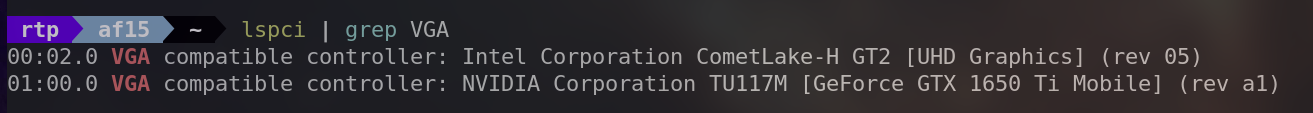`nvidia-smi`

It stands for "NVIDIA System Management Interface" It provides detailed information about the NVIDIA GPUs in your system, including utilization, temperature, memory usage and more.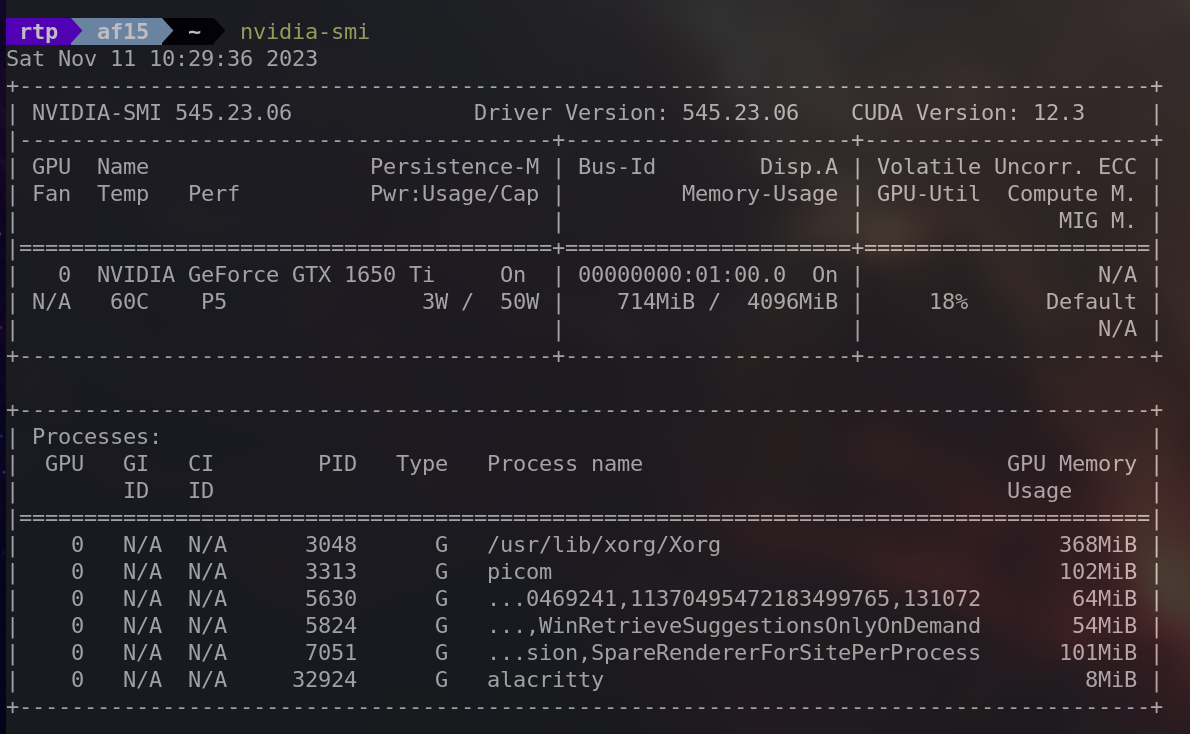`sudo lshw -C display`

The purpose is to provide detailed information about the display controllers in your system, including graphics cards.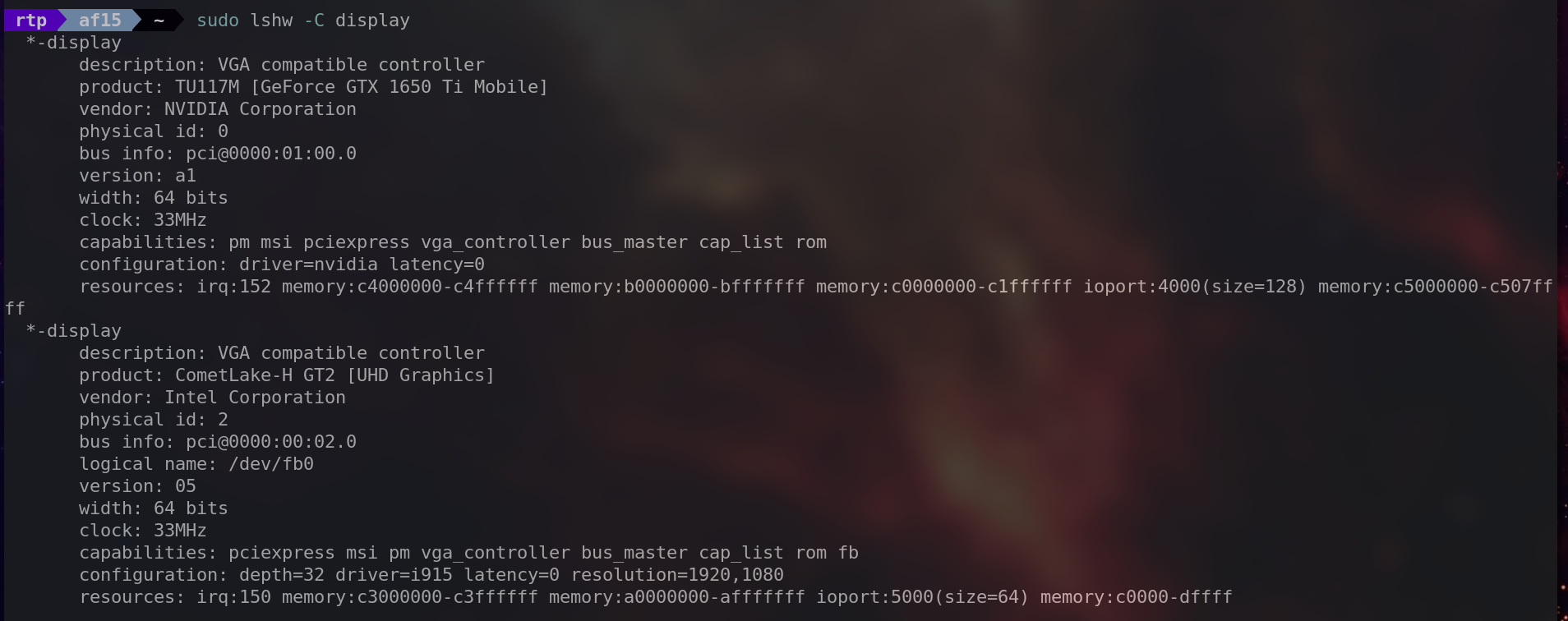`inxi -G`

This command provides information about the graphics subsystem, including details about the GPU and the display.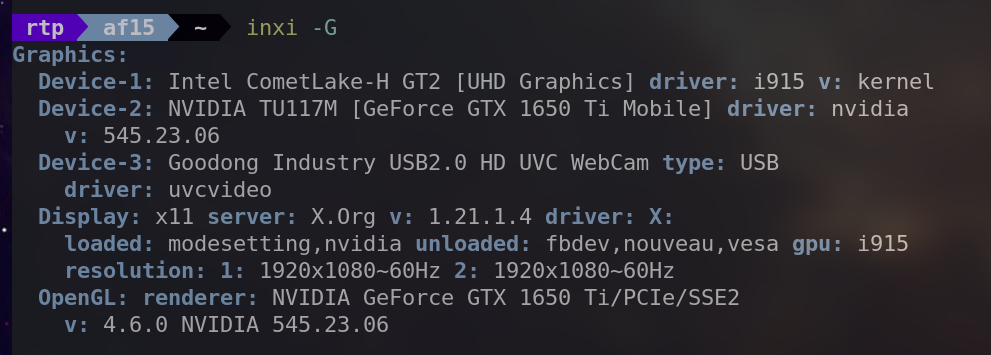`sudo hwinfo --gfxcard`

Its purpose is to obtain detailed information about the graphics cards in your system.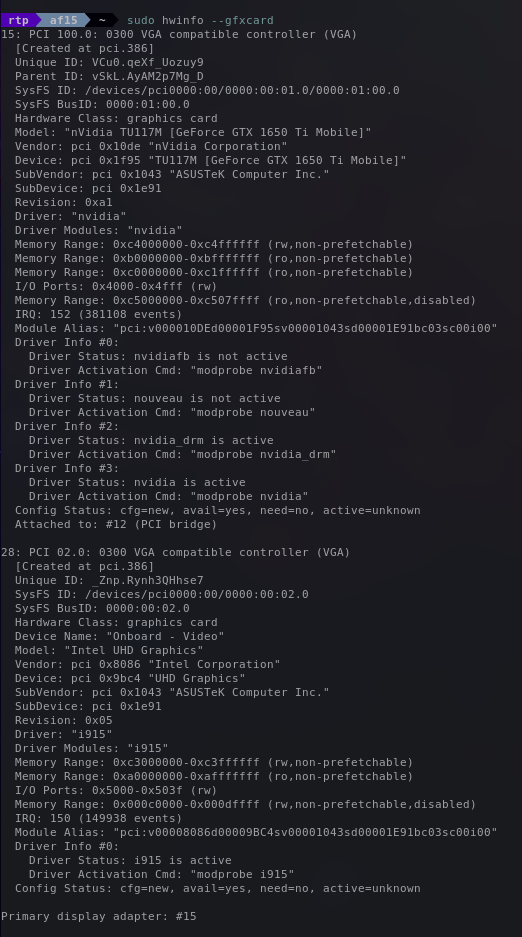### Get Started with the Cuda Framework

As we have installed the CUDA Framework, let's start executing operations that showcases its functionality.

A suitable problem to demonstrate the parallelization of GPUs is the Array addition problem.

Consider the following arrays:

• Array A = [1,2,3,4,5,6]

• Array B = [7,8,9,10,11,12]

• We need to store the sum of each element and store it in Array C.

• Like C = [1+7,2+8,3+9,4+10,5+11,6+12] = [8,10,12,14,16,18]

• If the CPU is to execute such operation, it would be executing the operation like the below code.

```#include <stdio.h>
int a[] = {1,2,3,4,5,6};
int b[] = {7,8,9,10,11,12};
int c;

int main() {
int N = 6;  // Number of elements

for (int i = 0; i < N; i++) {
c[i] = a[i] + b[i];
}

for (int i = 0; i < N; i++) {
printf("c[%d] = %d", i, c[i]);
}

return 0;
}
```

The previous method involves traversing the array elements one by one and performing the additions sequentially. However, when dealing with a substantial volume of numbers, this approach becomes sluggish due to its sequential nature.

To address this limitation, GPUs offer a solution by parallelizing the addition process. Unlike CPUs, which execute operations one after the other, GPUs can concurrently perform multiple additions.

For instance, the operations 1+7, 2+8, 3+9, 4+10, 5+11 and 6+12 can be executed simultaneously through parallelization with the assistance of a GPU.

Utilizing CUDA, the code to achieve this parallelized addition is as follows:

We will use a kernel file (.cu) for the demonstration.

Let's go through the code one by one.

```__global__ void vectorAdd(int* a, int* b, int* c)
{
c[i] = a[i] + b[i];
return;
}
```
• `__global__` specifier indicates that this function is a kernel function, which will be called on the GPU.

• `vectorAdd` takes three integer pointers (a, b, and c) as arguments, representing vectors to be added.

• `threadIdx.x` retrieves the index of the current thread (in a one-dimensional grid).

• The sum of the corresponding elements from vectors a and b is stored in vector c.

Now lets go through the main function.

Pointers `cudaA`, `cudaB` and `cudaC` are created to point to memory on the GPU.

```// Uses CUDA to use functions that parallelly calculates the addition
int main(){
int a[] = {1,2,3};
int b[] = {4,5,6};
int c[sizeof(a) / sizeof(int)] = {0};
// Create pointers into the GPU
int* cudaA = 0;
int* cudaB = 0;
int* cudaC = 0;
```

Using `cudaMalloc`, memory is allocated on the GPU for the vectors cudaA, cudaB, and cudaC.

```// Allocate memory in the GPU
cudaMalloc(&cudaA,sizeof(a));
cudaMalloc(&cudaB,sizeof(b));
cudaMalloc(&cudaC,sizeof(c));
```

The content of vectors a and b is copied from the host to the GPU using `cudaMemcpy`.

```// Copy the vectors into the gpu
cudaMemcpy(cudaA, a, sizeof(a), cudaMemcpyHostToDevice);
cudaMemcpy(cudaB, b, sizeof(b), cudaMemcpyHostToDevice);
```

The kernel function `vectorAdd` is launched with one block and a number of threads equal to the size of the vectors.

```// Launch the kernel with one block and a number of threads equal to the size of the vectors
vectorAdd <<<1, sizeof(a) / sizeof(a)>>> (cudaA, cudaB, cudaC);
```

The result vector `cudaC` is copied from the GPU back to the host.

```// Copy the result vector back to the host
cudaMemcpy(c, cudaC, sizeof(c), cudaMemcpyDeviceToHost);
```

We can then print the results as usual

```    // Print the result
for (int i = 0; i < sizeof(c) / sizeof(int); i++)
{
printf("c[%d] = %d", i, c[i]);
}

return 0;
}
```

For executing this code, we will use `nvcc` command.

We will get the output as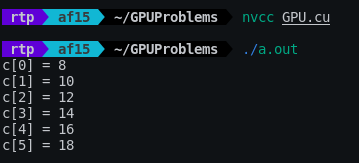Here's the full code for your reference.

#### Optimize Image Generation in Python Using the GPU

• This section explores the optimization of performance-intensive tasks, such as image generation, using GPU processing.

• Mandelbrot set is a mathematical construct that forms intricate visual patterns based on the behavior of specific numbers in a prescribed equation. Generating one is a resource intensive operation.

• In the following code snippet, you can observe the conventional method of generating a Mandelbrot set using CPU processing, which is slow.

```# Import necessary libraries
from matplotlib import pyplot as plt
import numpy as np
from pylab import imshow, show
from timeit import default_timer as timer

# Function to calculate the Mandelbrot set for a given point (x, y)
def mandel(x, y, max_iters):
c = complex(x, y)
z = 0.0j
# Iterate to check if the point is in the Mandelbrot set
for i in range(max_iters):
z = z*z + c
if (z.real*z.real + z.imag*z.imag) >= 4:
return i
# If within the maximum iterations, consider it part of the set
return max_iters

# Function to create the Mandelbrot fractal within a specified region
def create_fractal(min_x, max_x, min_y, max_y, image, iters):
height = image.shape
width = image.shape

# Calculate pixel sizes based on the specified region
pixel_size_x = (max_x - min_x) / width
pixel_size_y = (max_y - min_y) / height

# Iterate over each pixel in the image and compute the Mandelbrot value
for x in range(width):
real = min_x + x * pixel_size_x
for y in range(height):
imag = min_y + y * pixel_size_y
color = mandel(real, imag, iters)
image[y, x] = color

# Create a blank image array for the Mandelbrot set
image = np.zeros((1024, 1536), dtype=np.uint8)

# Record the start time for performance measurement
start = timer()

# Generate the Mandelbrot set within the specified region and iterations
create_fractal(-2.0, 1.0, -1.0, 1.0, image, 20)

# Calculate the time taken to create the Mandelbrot set
dt = timer() - start

# Print the time taken to generate the Mandelbrot set
print("Mandelbrot created in %f s" % dt)

# Display the Mandelbrot set using matplotlib
imshow(image)
show()
```

The above code produces the output in `4.07` seconds.• To make this faster, we can parallelize the code with GPU by using Numba library, Lets see how its done.

• We will import Just-In-Time compilation, CUDA for GPU acceleration, and other utilities from numba

```import numpy as np
from numba import jit, cuda, uint32, f8, uint8
from pylab import imshow, show
from timeit import default_timer as timer
```
• The `@jit` decorator signals Numba to perform Just-In-Time compilation, translating the Python code into machine code for improved execution speed.
```@jit
def mandel(x, y, max_iters):
c = complex(x, y)
z = 0.0j
for i in range(max_iters):
z = z*z + c
if (z.real*z.real + z.imag*z.imag) >= 4:
return i

return max_iters
```
• `mandel_gpu` is a GPU-compatible version of the mandel function created using cuda.jit. This allows the mandel logic to be offloaded to the GPU.
• This is done by using `@cuda.jit` decorator along with specifying the data types (f8 for float, uint32 for unsigned integer) for the function arguments.
• The `device=True` argument indicates that this function will run on the GPU.
```mandel_gpu = cuda.jit((f8, f8, uint32), device=True)(mandel)
```
• The mandel_kernel function is defined to be executed on the CUDA GPU. It is responsible for parallelizing the Mandelbrot set generation across GPU threads.
```@cuda.jit((f8, f8, f8, f8, uint8[:,:], uint32))
def mandel_kernel(min_x, max_x, min_y, max_y, image, iters):
height = image.shape
width = image.shape

pixel_size_x = (max_x - min_x) / width
pixel_size_y = (max_y - min_y) / height

startX, startY = cuda.grid(2)
gridX = cuda.gridDim.x * cuda.blockDim.x
gridY = cuda.gridDim.y * cuda.blockDim.y

for x in range(startX, width, gridX):
real = min_x + x * pixel_size_x
for y in range(startY, height, gridY):
imag = min_y + y * pixel_size_y
image[y, x] = mandel_gpu(real, imag, iters)
```
• Now, we can use the GPU-accelerated Mandelbrot set generation in the create_fractal_gpu function. This function allocates GPU memory, launches the GPU kernel (mandel_kernel), and copies the result back to the CPU.
```def create_fractal_gpu(min_x, max_x, min_y, max_y, image, iters):
# Step 1: Allocate GPU memory for the image
d_image = cuda.to_device(image)

# Step 2: Define the number of threads and blocks for GPU parallelization
blockspergrid = (blockspergrid_x, blockspergrid_y)

# Step 3: Measure the starting time
start = timer()

# Step 4: Launch the GPU kernel (mandel_kernel) to calculate the Mandelbrot set on the GPU
mandel_kernel[blockspergrid, threadsperblock](min_x, max_x, min_y, max_y, d_image, iters)

# Step 5: Wait for the GPU to finish its work (synchronize)
cuda.synchronize()

# Step 6: Measure the time taken by GPU processing
dt = timer() - start

# Step 7: Copy the results back from GPU memory to the CPU
d_image.copy_to_host(image)

# Step 8: Display the Mandelbrot set image
print("Mandelbrot created on GPU in %f s" % dt)
imshow(image)
show()
```

The above code gets executed in `0.0046 seconds`. Which is a lot faster the CPU Based code we had earlier.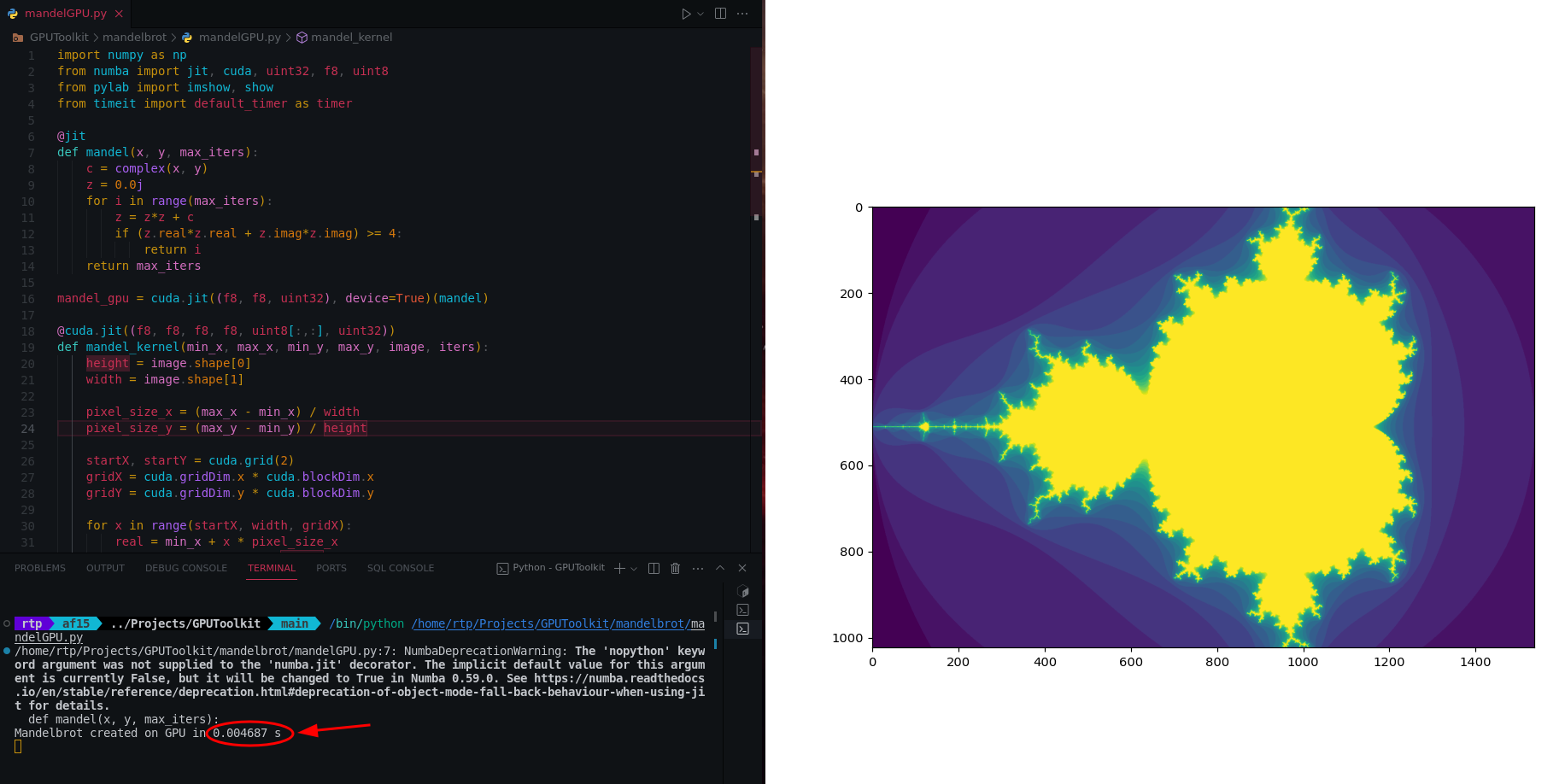Here's the full code for your reference.

#### Training a Cat VS Dog Neural Network Using the GPU

One of the hot topics we see nowadays is how GPUs are getting used in AI, So to demonstrate that we will be creating a neural network to differentiate between cats and dogs.

Prerequisites

• CUDA
• Tensorflow -> Can be installed via `pip install tensorflow[and-cuda]`

• We will use a data set of cats and dogs from kaggle

• Once you have downloaded it, Unzip them, organize the pictures of cats and dogs in the training folder into different subfolders, Like so.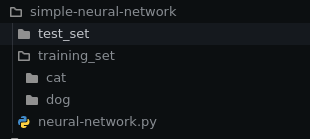This is the code we will use for training and using the Cat vs Dog Model.

The below code uses a convolutional neural network, you can read more details about it

Importing Libraries

• pandas and numpy for data manipulation.
• Sequential for creating a linear stack of layers in the neural network.
• Convolution2D, MaxPooling2D, Dense, and Flatten are layers used in building the Convolutional Neural Network (CNN).
• ImageDataGenerator for real-time data augmentation during training.
```import pandas as pd
import numpy as np
from keras.models import Sequential
from keras.layers import Convolution2D, MaxPooling2D, Dense, Flatten
from keras.preprocessing.image import ImageDataGenerator
```

Initializing the Convolutional Neural Network

```classifier = Sequential()
```

```train_datagen = ImageDataGenerator(
rescale=1./255,
shear_range=0.2,
zoom_range=0.2,
horizontal_flip=True
)
test_datagen = ImageDataGenerator(rescale=1./255)

training_set = train_datagen.flow_from_directory(
'./training_set',
target_size=(64, 64),
batch_size=32,
class_mode='binary'
)

test_set = test_datagen.flow_from_directory(
'./test_set',
target_size=(64, 64),
batch_size=32,
class_mode='binary'
)
```

Building the CNN Architecture

```classifier.add(Convolution2D(32, 3, 3, input_shape=(64, 64, 3), activation='relu'))
```

Compiling the model

```classifier.compile(optimizer='adam', loss='binary_crossentropy', metrics=['accuracy'])
```

Training the model

```classifier.fit(training_set, epochs=25, validation_data=test_set, validation_steps=2000)
classifier.save('trained_model.h5')
```

Once we have trained the model, The model is stored in a .h5 file using `classifier.save`

In the below code, we will use this `trained_model.h5` file to recognize cats and dogs.

```import numpy as np
import keras.utils as image

def predict_image(imagepath, classifier):
predict_modified = image.img_to_array(predict)
predict_modified = predict_modified / 255
predict_modified = np.expand_dims(predict_modified, axis=0)
result = classifier.predict(predict_modified)

if result >= 0.5:
prediction = 'dog'
probability = result
print("Probability = " + str(probability))
print("Prediction = " + prediction)
else:
prediction = 'cat'
probability = 1 - result
print("Probability = " + str(probability))
print("Prediction = " + prediction)

# Example usage
dog_image = "dog.jpg"

cat_image = "cat.jpg"
```

Let's see the output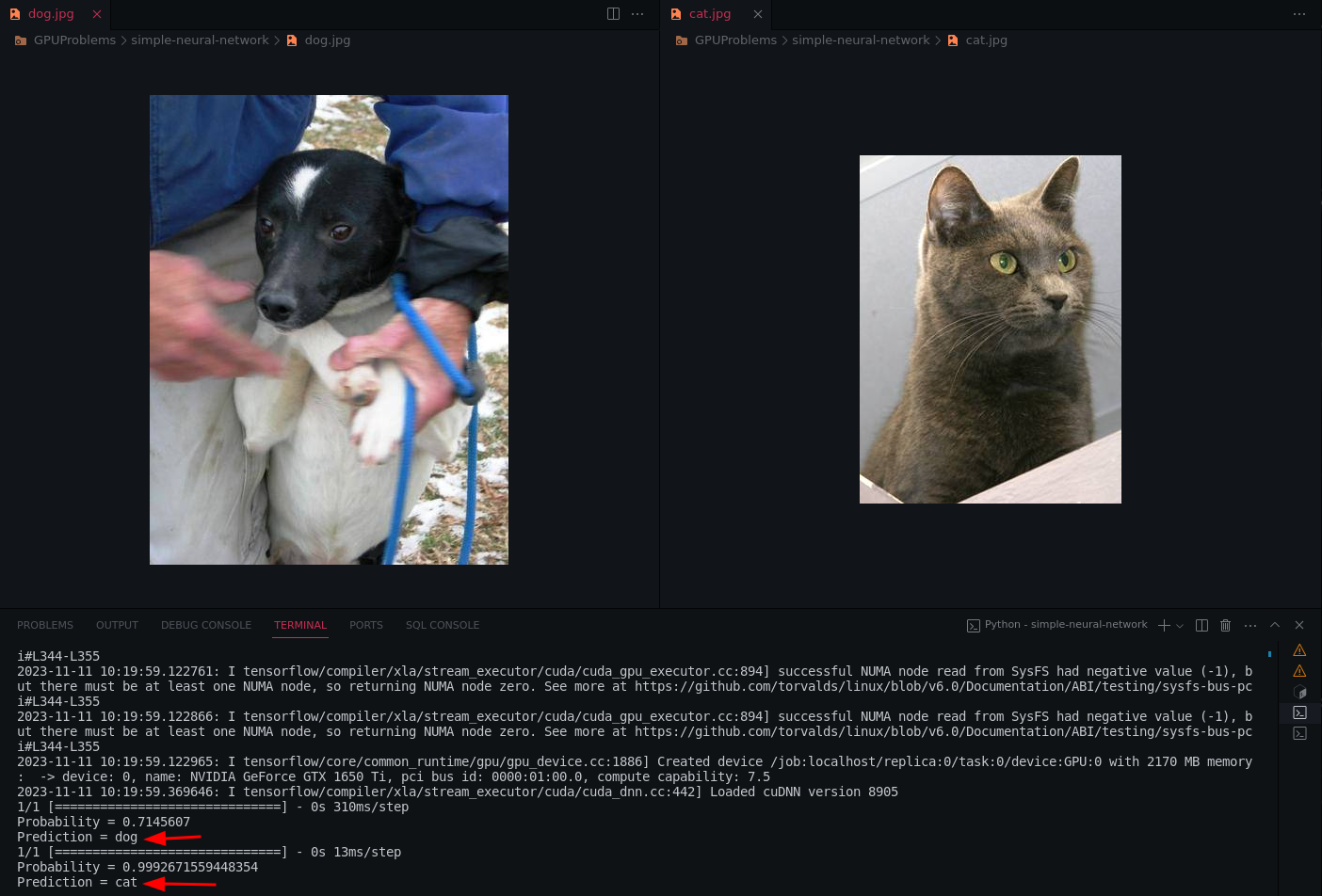Here's the full code for your reference

### Conclusion

In the upcoming AI age, GPUs are not a thing to be ignored, We should be more aware of its capabilities.

As we transition from traditional sequential algorithms to increasingly prevalent parallelized algorithms, GPUs emerge as indispensable tools that empower the acceleration of complex computations. The parallel processing prowess of GPUs is particularly advantageous in handling the massive datasets and intricate neural network architectures inherent to artificial intelligence and machine learning tasks.

Furthermore, the role of GPUs extends beyond traditional machine learning domains, finding applications in scientific research, simulations, and data-intensive tasks. The parallel processing capabilities of GPUs have proven instrumental in addressing challenges across diverse fields, ranging from drug discovery and climate modelling to financial simulations.

### Reference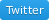Hackernews post